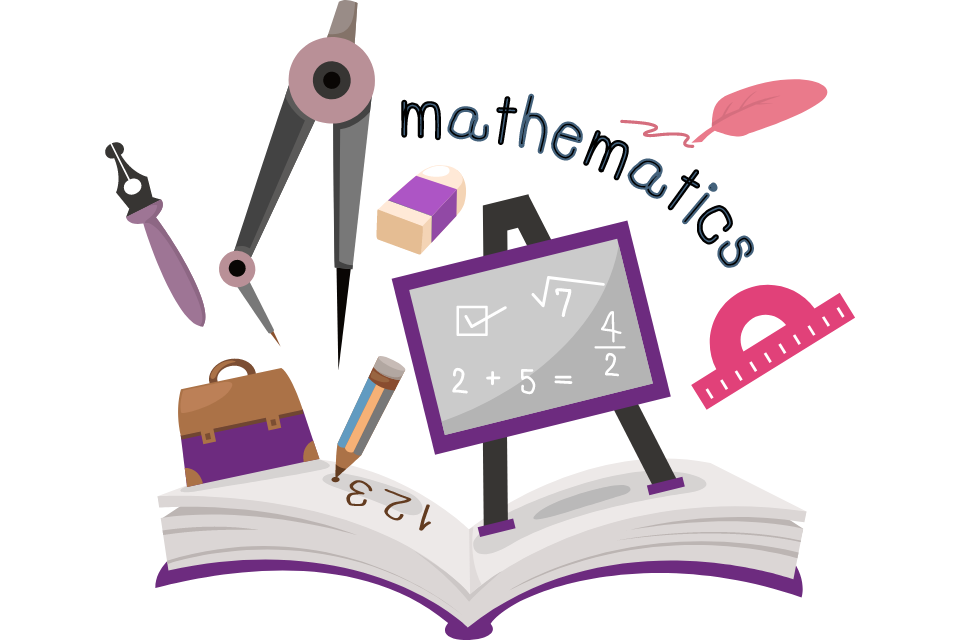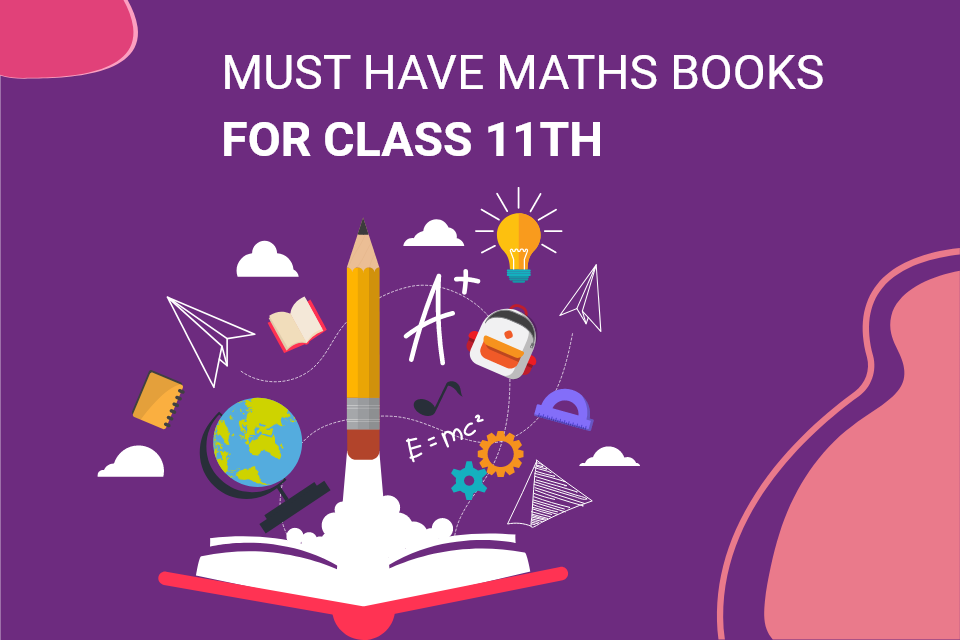# Maths Syllabus And Best Maths Books For Class 11 Students in IndiaPure mathematics is, in its way, the poetry of logical ideas, according to Albert Einstein.

It is essential for young minds to consider the statement. Nowadays, Maths seems to be the most difficult subject among the students; the primary reason is poor foundational base. For students who keep Maths in their higher secondary, it is mandated that they refer to the best Maths books for class 11th, which help them to clarify all their doubts. Maths is the abstract subject upon which mastery can only be done when you can relate it to real life. For example, the chapter Sets is one of the toughest chapters of Maths for class 11th students. At the same time, it serves as a fundamental part of today's lives. At present, this concept is widely used in almost every branch of mathematics. The ideas of relations and functions are defined using sets. The study of geometry, sequences, probability, and other subjects necessitates acquiring certain skills. German mathematician Ludwig Wittgenstein founded the set theory. There are several types of sets of important equations, and the students must have a clear understanding of these concepts to solve all the complex problems. Hence, referring to the good book will allow you to easily grasp the concept and provide various questions for your practice.

## Maths Syllabus For Class 11th In Detail

Before coming to any conclusion, you need to understand the syllabus deeply and know about the exam requirements. We all know that we have a multicultural nation in India with a unique education system including several national and state-level educational boards. There are four primary boards in our country: CBSE, ICSE, IB, and state boards. Considering the syllabus, it may slightly vary from board to board. Let us check the syllabus in detail.

### CBSE Class 11th Maths Syllabus:

• Unit I: Sets
• Unit II: Algebra
• Unit III: Coordinate Geometry
• Unit IV: Calculus
• Unit V: Mathematical Reasoning
• Unit VI: Statistics and Probability

### ICSE Class 11th Maths Syllabus:

• Chapter 1: Sets and Functions
• Chapter 2: Algebra
• Chapter 3: Coordinate Geometry
• Chapter 4: Calculus
• Chapter 5: Statistics & Probability
• Chapter 6: Conic Section
• Chapter 7: Introduction to three-dimensional Geometry
• Chapter 8: Mathematical Reasoning
• Chapter 9: Statistics
• Chapter 10: Correlation Analysis
• Chapter 11: Index Numbers and Moving Averages

The textbooks are prescribed to the class 11 students as per the guidelines of their respective boards. Students face numerous difficulties in solving the complex numerical questions as per the exam standard; hence it is essential to practice from some good reference books to overcome the problem. Let us check some of the best books for class 11th students

## Must-Have Maths Books For Class 11thIt's a well-known fact that the textbooks prescribed by the respective board are the best in exam preparation. But what if you are planning to sit for the national level competitive exams. Mugging up the fact of the prescribed textbook will not work. Hence, it is mandatory to take the help of the reference books, which helps make you understand the concepts clearly. The following are the list of best Maths books for class 11th:

• Senior Secondary School Mathematics- Class XI by R.S Aggarwal: The RS Aggarwal book gives you a thorough examination of all the topics you need to learn. This is why RS Aggarwal's book is one of the most popular choices for Maths preparation. This book will present you with a large number of practise problems, allowing you to apply the topics you've learned. Students should try to solve as many of the problems in this book as they can prepare for the board or theoretical examination and the JEE examination.

• Mathematics for Class XI by R.D Sharma: For class 11th students, RD Sharma (Ravi Dutt Sharma) is the most popular and demanding Math reference book. It is one of the greatest books since it covers all you need to know about each chapter in detail. It is made in accordance with CBSE and NCERT guidelines. Numerous solved questions may be found in RD Sharma's Class 11 book. The activities in each chapter have been revised to include additional visual examples and difficulty levels. In each chapter, all topics and terminology have been studied in-depth and, as well as presented with pertinent examples.

Other books such as Xam Idea Class 11th Maths, Together with class 11th Maths, and much more. However, only referencing the best book will not help you until you didn't receive guidance from the experts. If you fear Maths, then no worries, SSSi Onlne Tutoring Services is one of the best online learning platforms that offer the best class 11 Maths online tuition classes. The following are the benefits which you can avail of:

• Premium One-on-one online tuition for class 11
• Affordable fee structure